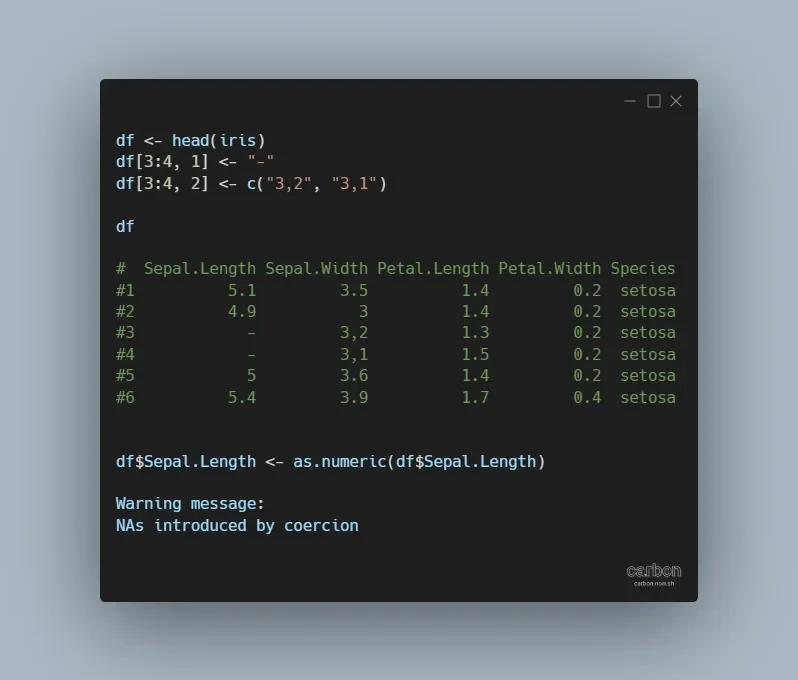# NAs introduced by coercion in R

If you see the warning NAs introduced by coercion in R, don’t panic. It is not necessarily bad, but you should understand if that is acceptable. This warning message usually appears by converting non-numerical values to numerical values with functions like as.numeric or as.integer. It may also appear by creating plots where the correct data type is essential.

For example, the data frame column may look numerical, but few records are not. Usually, that is the case when working with messy data.

Here is my data frame. As you can see, two columns contain non-numerical values. If you want to check data types, here is how to do that quickly.

```df <- head(iris)

df[3:4, 1] <- "-"

df[3:4, 2] <- c("3,2", "3,1")

df

#  Sepal.Length Sepal.Width Petal.Length Petal.Width Species
#1          5.1         3.5          1.4         0.2  setosa
#2          4.9           3          1.4         0.2  setosa
#3            -         3,2          1.3         0.2  setosa
#4            -         3,1          1.5         0.2  setosa
#5            5         3.6          1.4         0.2  setosa
#6          5.4         3.9          1.7         0.4  setosa```

If I create a basic scatter plot, the result might appear but with the warning messages like these.

```plot(df\$Sepal.Length, df\$Sepal.Width)

#Warning messages:
#1: In xy.coords(x, y, xlabel, ylabel, log) : NAs introduced by coercion
#2: In xy.coords(x, y, xlabel, ylabel, log) : NAs introduced by coercion```

## How to fix NAs introduced by coercion in R

The best situation is when you have to do nothing. For example, the first data frame column contains a symbol that represents the missing value.
After converting that column to a numeric one, the result is acceptable.

```df\$Sepal.Length <- as.numeric(df\$Sepal.Length)

#Warning message:
#NAs introduced by coercion

df

#  Sepal.Length Sepal.Width Petal.Length Petal.Width Species
#1          5.1         3.5          1.4         0.2  setosa
#2          4.9           3          1.4         0.2  setosa
#3           NA         3,2          1.3         0.2  setosa
#4           NA         3,1          1.5         0.2  setosa
#5          5.0         3.6          1.4         0.2  setosa
#6          5.4         3.9          1.7         0.4  setosa```

The second situation is when you don’t want to get the NAs. As you can see, the second data frame column contains an incorrect decimal separator. In this scenario, you want to replace the incorrect decimal separator with the correct one before converting it to numeric.

```df\$Sepal.Width <- as.numeric(gsub(",", ".", df\$Sepal.Width))

df

#  Sepal.Length Sepal.Width Petal.Length Petal.Width Species
#1          5.1         3.5          1.4         0.2  setosa
#2          4.9         3.0          1.4         0.2  setosa
#3           NA         3.2          1.3         0.2  setosa
#4           NA         3.1          1.5         0.2  setosa
#5          5.0         3.6          1.4         0.2  setosa
#6          5.4         3.9          1.7         0.4  setosa```

Sometimes missing values may lead to further problems.

Here are some other things that can help you to deal with NA values in R. For example, you can check if the column contains missing values or replace NA with something necessary.

If you want to replace NA in a certain range, here is how to do that. In some situations, you want to fill values down like this.

Thank you for reading this post and I hope it helps in your work in R programming.

Categories R